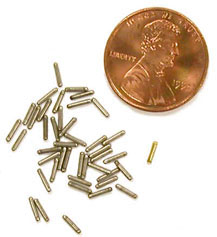## Friday, February 19, 2016

### The Sievert Integral

In Section 17.11 of Intermediate Physics for Medicine and Biology, Russ Hobbie and I discuss Brachytherapy.
Brachytherapy (brachy means short) involves implanting directly in a tumor sources for which the radiation falls off rapidly with distance because of attenuation, short range, or 1/r2. Originally the radioactive sources (seeds) were implanted surgically, resulting in high doses to the operating room personnel. In the afterloading technique, developed in the 1960s, hollow catheters are implanted surgically and the sources inserted after the surgery. Remote afterloading, developed in the 1980s, places the sources by remote control, so that only the patient receives a radiation dose.Bracytherapy sources.
Often brachytherapy is performed by implanting a source of radiation formed as a line. Below is a new homework problem for calculating the dose of radiation assuming a small line source. You will do the calculation with and without a shield surrounding the source.

Section 17.11

Problem 56 ½. Brachytherapy is often performed using a radioactive source shaped as a line of length L having a total cumulated activity Ã and a mean energy emitted per unit cumulated activity Δ. Assume Eq. 17.50 describes the specific absorbed fraction Φ in the surrounding tissue having an energy absorption coefficient μen and density ρ

(a) Calculate the dose D a distance h away from the center of the line source (assume h is much less than both L and 1/μen). Let x indicate the position along the source, and set x = 0 at the center, so r2 = x2 + h2. The total dose is an integral over the length of the source, which has a cumulated activity per unit length Ã/L. Evaluate this integral using the substitution x = h tanθ. In the limits of integration, ignore end effects by letting L extend to infinity. You may need the trigonometric relationships d(tanθ)/dθ = sec2θ and 1 + tan2θ = sec2θ.

(b) Repeat the calculation in part (a), except add a coaxial cylindrical shield of thickness b surrounding the line source, made of a material having an absorption coefficient μatten. The dose from a small section of the source is now attenuated by an additional factor of exp(−μattenbsecθ). Justify the factor of secθ in the exponential. Show that the dose can now be written as the result from part (a) times 2/π times a definite integral, called the Sievert integral. Derive an expression for the Sievert integral.

(c) Make a drawing that indicates the physical meaning of h, b, x, r, L, and θ. Explain why the dose is inversely proportional to L.
The Sievert integral is analyzed and tabulated in the Handbook of Mathematical Functions with Formulas, Graphs, and Mathematical Tables by Abramowitz and Stegun. It can be generalized to include end effects. The integral is named after Rolf Sievert, the Swedish medical physicist who is honored by the SI unit for equivalent dose: the sievert.